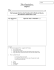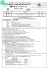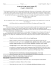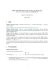Problem Set 3

Transcription

Problem Set 3
COMP 360: Algorithm Design
Instructor: Yang Cai
Problem Set 3
Due: Mar 16, 2015
General Rules: Follow the collaboration policy. In particular, each of you
should write your own solution even if you have collaborated with your classmates. In solving these questions you may consult books but you may not
search on the web for solutions. You should drop your solutions in the assignment drop-off box located in the Trottier Building. No late assignments
accepted.
1. (10 pts) Number Partition.
Subset Sum is the following problem: given natural numbers w1 , . . . , wn , and a target number
W, is there a subset of {w1 , . . . , wn } that adds up to precisely W?
Use the fact that Subset Sum is NP-complete to solve p518, Qu. 8.26.
2. (15 pts) Dominating Set. p519, Qu. 8.29.
3. (15 pts) Undirected Feedback Set. p520, Qu. 8.31. Notice that no double edges are allowed. That
is, between any two vertices u and v, only one edge (u, v) is allowed in the graph G.
4. (15 pts) Influence Maximization. p524, Qu. 8.34.
5. (20 pts) Prove that the following problem is NP-complete:
• Input: A number k, and a formula ϕ in conjunctive normal form.
• Output: Is there a truth assignment that satisfies ϕ and assigns False to exactly k variables?
What happens if in the above problem we replace k with the fixed number 10?
1-1

Exam 3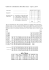10.1\\\-10.3 review ans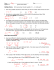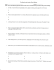homework 4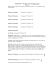Automata Theory Sample Midterm Exam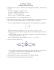Math 0120 Examination #3 Sample Name (Print)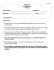Math 30G – Calculus – Sample Final Exam Fall 2012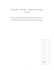Assignment 2 the 1.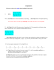Blank PDF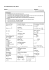3w 3 t5+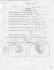Sample Exam 3 - People Pages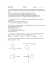2 for 1 Dinner Menu - Club Regent Casino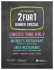Name _____Mr. Perfect________________________________ Date __F 14_______ n l of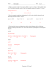Cover Sheet! EXAM2 PRINT your name legibly on the line below.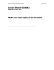Questions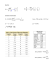Exam 3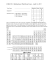Problem Set 4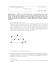AP Chemistry Summer Work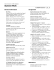The Outsiders: - Mr. Sturgeon`s Wonderful World of English